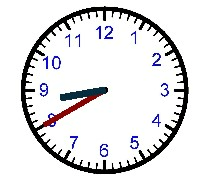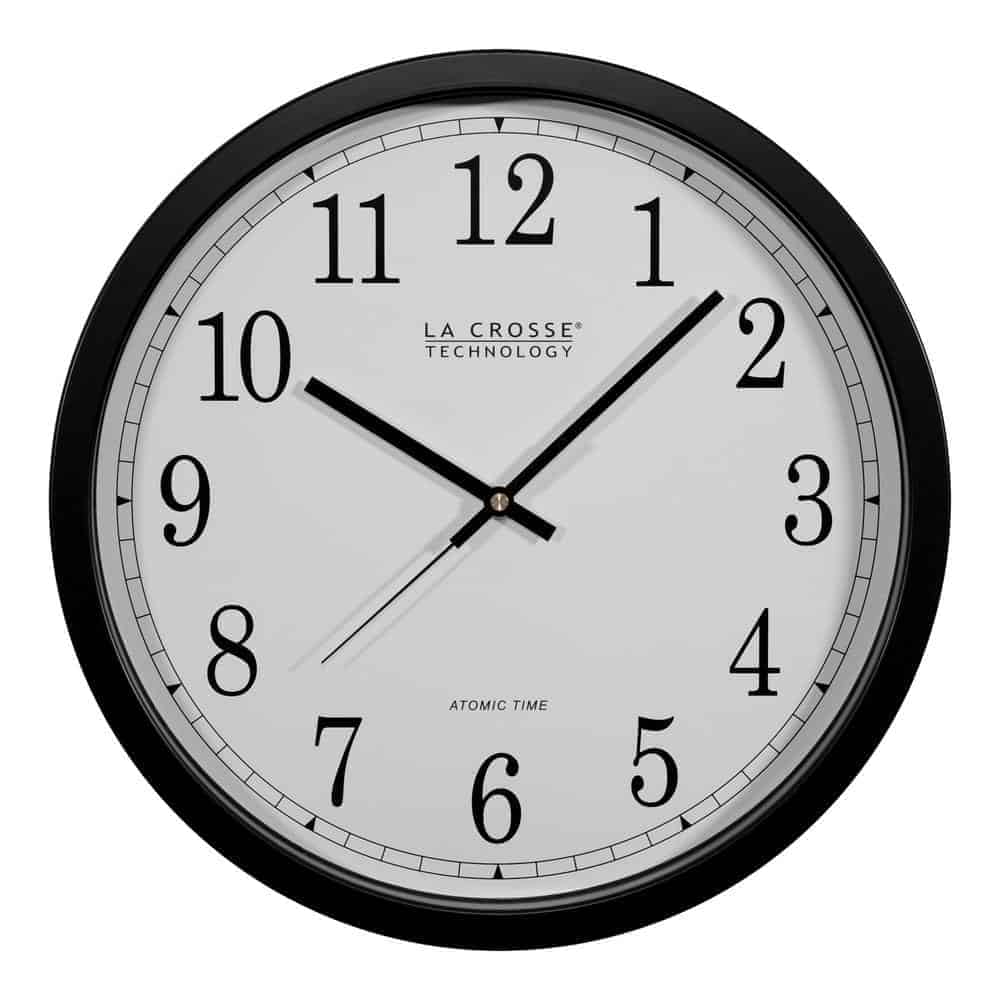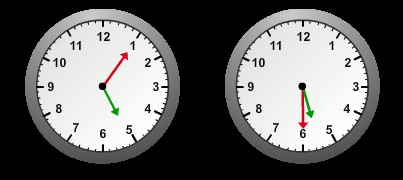Random
100

10 x 2

20
100

12 Divided By 4

3

100

What is 57 rounded to the nearest ten?

What is 60?

100

What is the time on the analog clockWhat is 8:40 am/pm

100
Equal parts of a whole

What is a fraction?

200

3x8

24

200

20 Divided By 5

4

200

The digit in the tens place 678.

What is 7?

200

How much time passes?15 minutes

200

The area of a rectangle with a length of 5 ft. and a width of 3 ft.

What is 15 square feet?

300

7x6

42

300

27 Divided by 3

9
300

What is 625 rounded to the nearest hundred?

What is 700?

300It's 10:08 am/pm

300

Would you measure the water in a bathtub in L or mL?

What is L?

400

3x12

36

400

100 divided by 10

10

400

What is 825 rounded to the nearest ten?

What is 830?

400

How long is piano class?25 minutes

400

Does a paperclip weigh about a g or a kg?

What is a gram?

500

700 x 8

5,600

500

540 divided by 9

60

500

What is 7,852 rounded to the nearest hundred?

What is 7,900?

500

Tim and Bob play outside for 1 hr. 15 min. If they started playing at 2:15, what time did they end?

What is 3:30?

500

If there are 8 puppies in a litter and 5 have spots, what fraction do not have spots?

What is 3/8?

Click to zoom